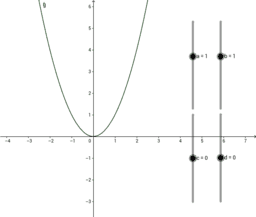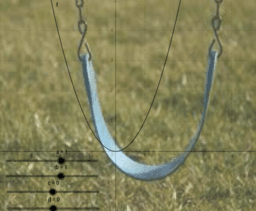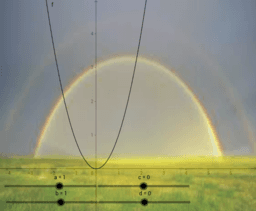# Algebra 2-Parent Functions

includes: an introductory applet in which viewers can explore the effects of changing the parent function f(x)=x^2 by changing the constants in the equation g(x)=a(bx+c)^2+d; three images of parabolic figures which will solidify viewers' understanding how to adjust a parent function to fit a certain curve
•### Graphing from the (Quadratic) Parent Function

•### Swing Problem

•### Rainbow Problem

•